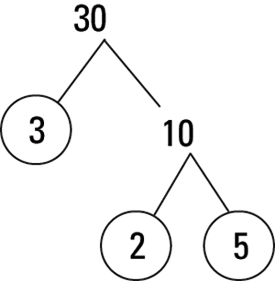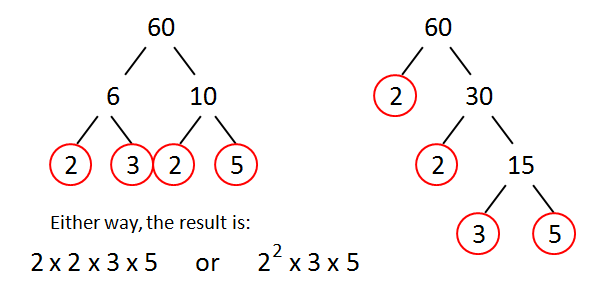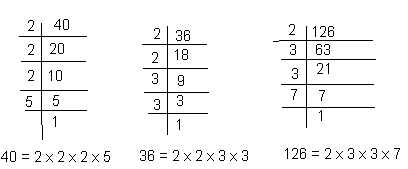# Prime factors:

The factors of any number which is a prime number are prime factors of that number.

Or simply,

Prime numbers when multiplied together gives a new number then the prime numbers are said to be prime factors of the new number.

Example: Suppose 21 is any number obtained by multiplying two prime numbers 3 and 7. Then 3 and 7 are said to be the prime factor of 21.

## How to find prime factors?

There are two methods for finding the prime factor:

### 1.    Factor tree method

Factor tree method is very simple and unique method to find the prime factor of any number. This tree continues to expand its branches until there is no factors left any more.

Prime factors of 24 by factor tree method:• The first branch contains 6 x 4
• Now, branch of 4 expanded to 2 x 2
• Again branch of 6 expanded to 2 x 3

Now no more factors are possible. Thus the prime factor of 24 are 2 and 3 but we write prime factorization as 2 x 2 x 2 x 3.

Prime factors of 30 by factor tree method:• The first branch contains 10 x 3.
• Now, the branch of 10 expanded to 2 x 5.

Now no more prime factor is possible. Thus the prime factor of 30 are 2, 3 and 5 but we write prime factorization as 2 x 3 x 5.

Prime factors of 60 by factor tree method:• The first branch contains 30 x 2
• Now, branch of 30 expanded to 6 x 5
• Again branch of 6 expanded to 2 x 3

Now no more prime factors are possible. Thus the prime factor of 60 are 2, 3 and 5 but we write prime factorization as 2 x 2 x 3 x 5.

### 2.    Repeated Division: the Actual factorization method

This method is similar to taking LCM of any number. First, we will divide it by the smallest possible prime number and then it continues till no more factors left.Thus we show that after each step we get the prime factors and the value of the number decreases to the lowest prime number.

## List of prime factors :

Here we have provided the list of factors to make it easy for you to understand.

 Numbers Prime Factorization Prime factor 24 2 × 2 × 2 × 3 2, 3 30 2 × 3 × 5 2, 3, 5 60 2 × 2 × 3 × 5 2, 3, 5 42 2 × 3 × 7 2, 3, 7 12 2 × 2 × 3 2, 3